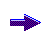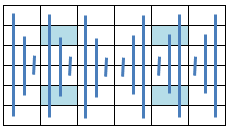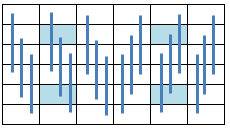Office Applications and Entertainment, Magic SquaresIndex About the Author

14.0    Special Magic Squares, Prime Numbers

14.4.30 Rectangular Compact Magic Squares (6 x 6)

In previous sections the property ‘Compact’ - defined as: each 2 x 2 Sub Square sums to 4 * s1 / n - has been introduced and applied for Prime Number Magic Squares of order 4 and 6.

For order 6 Magic Squares, Rectangular Compact can be defined as: Each 2 x 3 Sub Rectangle sums to the Magic Sum.

14.4.31 Partly Rectangular Compact

A Magic Square can be referred to as Partly Rectangular Compact, when only the six non overlapping Sub Rectangles (2 x 3) sum to the Magic Sum:

 a(1) a(2) a(3) a(4) a(5) a(6) a(7) a(8) a(9) a(10) a(11) a(12) a(13) a(14) a(15) a(16) a(17) a(18) a(19) a(20) a(21) a(22) a(23) a(24) a(25) a(26) a(27) a(28) a(29) a(30) a(31) a(32) a(33) a(34) a(35) a(36)

Partly Rectangular Compact Magic Squares can be described by following set of linear equations:

```a(31) =   s1 - a(32) - a(33) - a(34) - a(35) - a(36)
a(28) =   s1 - a(29) - a(30) - a(34) - a(35) - a(36)
a(25) =   s1 - a(26) - a(27) - a(28) - a(29) - a(30)
a(19) =   s1 - a(20) - a(21) - a(22) - a(23) - a(24)
a(16) =   s1 - a(17) - a(18) - a(22) - a(23) - a(24)
a(13) =   s1 - a(14) - a(15) - a(16) - a(17) - a(18)
a(11) = 4*s1 + a(12) + a(17) + 2*a(18) - 2*a(19) - 2*a(20) - 3*a(21) - a(22) - a(23) - 2*a(25) +
- 3*a(26) - 2*a(27) - a(28) - a(29) - 3*a(31) - 2*a(32) - 2*a(33) - a(34) - a(35)
a( 8) =  (- a( 9) - a(10) - 2*a(12) - a(14) - 2*a(15) - a(17) - 2*a(18) + 2*a(19) + a(20) + 2*a(21) +
+ a(23) + 2*a(25) + 2*a(26) + a(27) + a(28) + 2*a(31) - 2*a(36))/2
a( 7) =   s1 - a( 8) - a( 9) - a(10) - a(11) - a(12)
a( 6) =   s1 - a(12) - a(18) - a(24) - a(30) - a(36)
a( 5) =   s1 - a(11) - a(17) - a(23) - a(29) - a(35)
a( 4) =   s1 - a(10) - a(16) - a(22) - a(28) - a(34)
a( 3) =   s1 - a( 9) - a(15) - a(21) - a(27) - a(33)
a( 2) =   s1 - a( 8) - a(14) - a(20) - a(26) - a(32)
a( 1) =   s1 - a( 7) - a(13) - a(19) - a(25) - a(31)
```

which could be incorporated in a guessing routine to produce subject Magic Squares. However such a routine is not very feasible due to the high number of independent variables (21 ea).

Fortunately the property defined above is shared by following Magic Squares:

as deducted and discussed in Sections Section 14.4.10 thru Section 14.4.12.

14.4.32 Rectangular Compact

Rectangular Compact Magic Squares can be described by following set of linear equations:

```a(31) =      s1 - a(32) - a(33) - a(34) - a(35) - a(36)
a(28) =      s1 - a(29) - a(30) - a(34) - a(35) - a(36)
a(27) =           a(30) - a(33) + a(36)
a(26) =           a(29) - a(32) + a(35)
a(25) =          -a(29) - a(30) + a(32) + a(33) + a(34)
a(22) =         - a(23) - a(24) + a(34) + a(35) + a(36)
a(21) =           a(24) + a(33) - a(36)
a(20) =           a(23) + a(32) - a(35)
a(19) =      s1 - a(23) - a(24) - a(32) - a(33) - a(34)
a(16) =      s1 - a(17) - a(18) - a(34) - a(35) - a(36)
a(15) =           a(18) - a(33) + a(36)
a(14) =           a(17) - a(32) + a(35)
a(13) =           a(17) - a(18) + a(32) + a(33) + a(34)
a(12) =  7*s1/3 - a(17) - 2 * a(18) - a(30) - 2 * a(32) - 2 * a(34) - a(35) - 4 * a(36)
a(11) =    s1/3 - a(29)
a(10) = -8*s1/3 + a(17) + 2 * a(18) + a(29) + a(30) + 2 * a(32) + 3 * a(34) + 2 * a(35) + 5 * a(36)
a( 9) =  7*s1/3 - a(17) - 2 * a(18) - a(30) - 2 * a(32) + a(33) - 2 * a(34) - a(35) - 5 * a(36)
a( 8) =    s1/3 - a(29) + a(32) - a(35)
a( 7) = -5*s1/3 + a(17) + 2 * a(18) + a(29) + a(30) + a(32) - a(33) + a(34) + a(35) + 4 * a(36)
a( 6) = -4*s1/3 + a(17) + a(18) - a(24) + 2 * a(32) + 2 * a(34) + a(35) + 3 * a(36)
a( 5) =  2*s1/3 - a(17) - a(23) - a(35)
a( 4) =  5*s1/3 - a(18) + a(23) + a(24) - 2 * a(32) - 3 * a(34) - a(35) - 4 * a(36)
a( 3) = -4*s1/3 + a(17) + a(18) - a(24) + 2 * a(32) - a(33) + 2 * a(34) + a(35) + 4 * a(36)
a( 2) =  2*s1/3 - a(17) - a(23) - a(32)
a( 1) =  2*s1/3 - a(18) + a(23) + a(24) - a(32) + a(33) - a(34) - 3 * a(36)
```

which can be incorporated in a routine to generate subject Rectangular Compact Magic Squares (ref. MgcSqr6103).

Following consequential symmetry is worth to be noticed:

a( 8) = s1 / 3 - a(26)
a(11) = s1 / 3 - a(29)

and the reason that order 6 Rectangular Compact Magic Squares can't be Diagonal Symmetric.

Attachment 6.10.3 shows for miscellaneous Magic Sums (24 ea) the first occurring Prime Number Rectangular Compact Magic Square.

It can be noticed that for some of the Magic Sums, the first occuring solution is Row Symmetric and consequently Pan Magic, as discussed in Section 14.4.34 below.

Each square shown corresponds with numerous squares for the applicable Magic Sum and variable values {ai}.

14.4.33 Rectangular Compact, Axial Symmetric

Although Rectangular Compact Magic Squares can't be Diagonal Symmetric they can be Axial Symmetric.

When the equations defining Axial Symmetry as illustrated below:are added to the equations describing a Rectangular Compact Magic Square of the sixth order (Section 14.4.32), the resulting Magic Square is described by following set of linear equations:

```a(31) =   s1 - a(32) - a(33) - a(34) - a(35) - a(36)
a(28) =   s1 - a(29) - a(30) - a(34) - a(35) - a(36)
a(27) =        a(30) - a(33) + a(36)
a(26) =        a(29) - a(32) + a(35)
a(25) =      - a(29) - a(30) + a(32) + a(33) + a(34)
a(23) = - s1 - 2 * a(24) + 2 * a(32) + 2 * a(34) + a(35) + 4 * a(36)
a(22) =   s1 + a(24) - 2 * a(32) - a(34) - 3 * a(36)
a(21) =        a(24) + a(33) - a(36)
a(20) = - s1 - 2 * a(24) + 3 * a(32) + 2 * a(34) + 4 * a(36)
a(19) = 2*s1 + a(24) - 3 * a(32) - a(33) - 3 * a(34) - a(35) - 4 * a(36)
```
 a(18) = s1/3 - a(24) a(17) = s1/3 - a(23) a(16) = s1/3 - a(22) a(15) = s1/3 - a(21) a(14) = s1/3 - a(20) a(13) = s1/3 - a(19) a(12) = s1/3 - a(30) a(11) = s1/3 - a(29) a(10) = s1/3 - a(28) a( 9) = s1/3 - a(27) a( 8) = s1/3 - a(26) a( 7) = s1/3 - a(25) a(6) = s1/3 - a(36) a(5) = s1/3 - a(35) a(4) = s1/3 - a(34) a(3) = s1/3 - a(33) a(2) = s1/3 - a(32) a(1) = s1/3 - a(31)

which can be incorporated in a routine to generate subject Rectangular Compact Axial Symmetric Magic Squares (ref. MgcSqr6104).

Attachment 6.10.4 shows for miscellaneous Magic Sums (24 ea) the first occurring Prime Number Rectangular Compact Axial Symmetric Magic Square.

Each square shown corresponds with numerous squares for the applicable Magic Sum and variable values {ai}.

14.4.34 Rectangular Compact, Row Symmetric, Pan Magic

When the equations defining Row Symmetry as illustrated below:are added to the equations describing a Rectangular Compact Magic Square of the sixth order (Section 14.4.32), the resulting Magic Square can be described by following set of linear equations:

```a(32) =  s1/2 - a(34) - a(36)
a(31) =  s1/2 - a(33) - a(35)
a(28) =  s1   - a(29) - a(30) - a(34) - a(35) - a(36)
a(27) =         a(30) - a(33) + a(36)
a(26) = -s1/2 + a(29) + a(34) + a(35) + a(36)
a(25) =  s1/2 - a(29) - a(30) + a(33) - a(36)
a(22) =       - a(23) - a(24) + a(34) + a(35) + a(36)
a(21) =         a(24) + a(33) - a(36)
a(20) =  s1/2 + a(23) - a(34) - a(35) - a(36)
a(19) =  s1/2 - a(23) - a(24) - a(33) + a(36)
```
 a(18) = s1/3 - a(36) a(17) = s1/3 - a(35) a(16) = s1/3 - a(34) a(15) = s1/3 - a(33) a(14) = s1/3 - a(32) a(13) = s1/3 - a(31) a(12) = s1/3 - a(30) a(11) = s1/3 - a(29) a(10) = s1/3 - a(28) a( 9) = s1/3 - a(27) a( 8) = s1/3 - a(26) a( 7) = s1/3 - a(25) a(6) = s1/3 - a(24) a(5) = s1/3 - a(23) a(4) = s1/3 - a(22) a(3) = s1/3 - a(21) a(2) = s1/3 - a(20) a(1) = s1/3 - a(19)

and appears to be Pan Magic as well.

The equations above can be incorporated in a routine to generate subject Rectangular Compact Row Symmetric Pan Magic Squares (ref. MgcSqr6105).

Attachment 6.10.5 shows for miscellaneous Magic Sums (24 ea) the first occurring Prime Number Rectangular Compact Row Symmetric Pan Magic Square.

Each square shown corresponds with numerous squares for the applicable Magic Sum and variable values {ai}.

14.4.35 Summary

The obtained results regarding the miscellaneous types of order 6 Prime Number Rectangular Compact Magic Squares as deducted and discussed in previous sections are summarized in following table:

 Type Characteristics Subroutine Results Rect. Compact General Axial Symmetric Row   Symmetric, Pan Magic
 Comparable routines as listed above, can be used to generate Prime Number Magic Squares of order 7, which will be described in following sections.